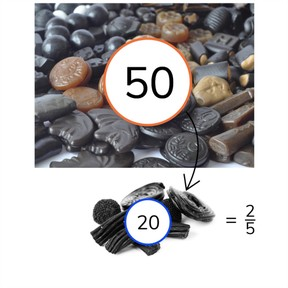Determine a portion with a fraction

# Determine a portion with a fraction

I can determine a portion of a set by using a fraction.

No account needed.8,000 schools use Gynzy92,000 teachers use Gynzy1,600,000 students use Gynzy

## General

Students can determine a portion of a total with a fraction.

## Standards

CCSS.MATH.CONTENT.4.NF.A.1
CCSS.MATH.CONTENT.5.NF.B.4

## Relevance

It is important to learn how to do this so you can use a fraction to determine which portion of a total you have.

## Introduction

Students are asked to name the fractions represented by the fraction bar.

## Development

Discuss briefly with students that a fraction is made of a numerator and a denominator. Explain that if you want to determine a portion with a fraction you must first determine what 1 unit of the total is. Show how to calculate this by dividing the total by the numerator. So 64 ÷ 8 = 8. Next you want to know how many this is in relation to the amount of units you have. You multiply the amount of one unit (so 8) by the numerator: 8 x 3 = 24. Repeat these steps with the problem of 6//9 if 81. With the next two problems, check that students are able to determine the portion with a fraction with the visual support of a fraction bar. Next show students how to determine 1//5 of 25. You only need to divide by the denominator, because you already know that you need 1 unit of the total. With 2//4 of 16, you divide by the denominator and multiply by the numerator. Ask students to solve the next two problem without visual support. There is space on the interactive whiteboard to write the steps to solving the problems, should you decide to solve them as a class. Next explain how to solve the fraction story problems. Emphasize that you first must determine which numbers are important before solving the fraction word problem.

Check that students are able to determine a portion with a fraction by asking the following question:
- Which steps do you take to solve a portion of a total using a fraction?

## Guided practice

Students are given problems with visual support, then without visual support and finally are given story problems to solve.

## Closing

Discuss the importance of determining a portion of a a total with a fraction in everyday life. To close, ask students to determine which of the given children has the most candies.

## Teaching tips

For students who have difficulty determining a portion of a total using a fraction, you can first practice determining simple fractions. Show them how to divide the total by the denominator to see how many are in one unit, or base fraction.

### The online teaching platform for interactive whiteboards and displays in schools

• Save time building lessons

• Manage the classroom more efficiently

• Increase student engagement